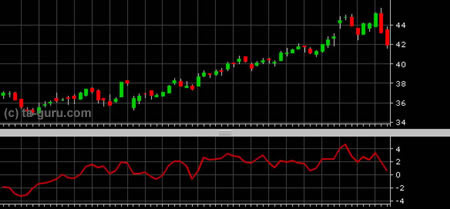Chart Pro online
User name (email):

 Recently used

 Forex

 Indices

# Momentum

Momentum belongs to a group of oscillators. It measures price changes from one period to next. The formula for calculating momentum is:

Momentum = CLOSE (i) - CLOSE (i-N)

Momentum is always a step ahead of changes in prices. So it belongs to leading indicators.

Momentum is actually measuring the rate of ascent or descent. If value of momentum is positive it indicates presence of an upward trend. As momentum grows it means that the trend is becoming stronger. If value falls, but still is above zero, it means that still upward trend is present, just losing its power. For the downward trend opposite is true. Downward trend is present when momentum is negative and its power is greater if momentum is decreasing.

The biggest drawback of this indicator is the absence of boundaries. It was mentioned in chapter about technical indicators that each oscillator has defined values between its values are oscillating, usually 0 and 100. But the momentum doesn't have it. That's why some technicians are drawing boundaries based on values of this indicator in the past and correcting them dynamically.Momentum

Interpretation

Values of momentum indicator are oscillating around zero. Buying signal is generated when line of momentum crosses above zero line. Selling signal is generated in the opposite case, when it crosses below zero line.

There is another interpretation of momentum: selling signal is generated when the value of indicator begins to fall after the generated local maximum, and buying when it begins to grow after a local minimum.

Momentum can also be used for observation of divergence. So if price falls to new low, but momentum fails to reach new low, downward trend is losing its power and we could expect trend reversal in the near future. Similar goes for upward trend: if momentum fails to reach new high when price hits new high, upward trend is losing its power.

Example